# MSBSHSE Solutions For SSC (Class 10) Maths Part 2 Chapter 4 - Geometric Constructions

MSBSHSE Solutions For SSC (Class 10) Maths Part 2 Chapter 4 – Geometric Constructions are given here with detailed steps of constructions and clear diagrams. BYJU’S provides you precise solutions, which are prepared by our dedicated specialists. All solutions from MSBSHSE Solutions For SSC (Class 10) Maths Part 2 Chapter 4 are provided here for free. These solutions help students to familiarize themselves with geometric constructions of triangles, similar to a given circle. This chapter also deals with construction of tangents to a circle using the centre of the circle and without using the centre of the circle. Solutions are available in PDF format on our website.

## Download the PDF of Maharashtra Solutions For SSC Maths Part 2 Chapter 4 Geometric Constructions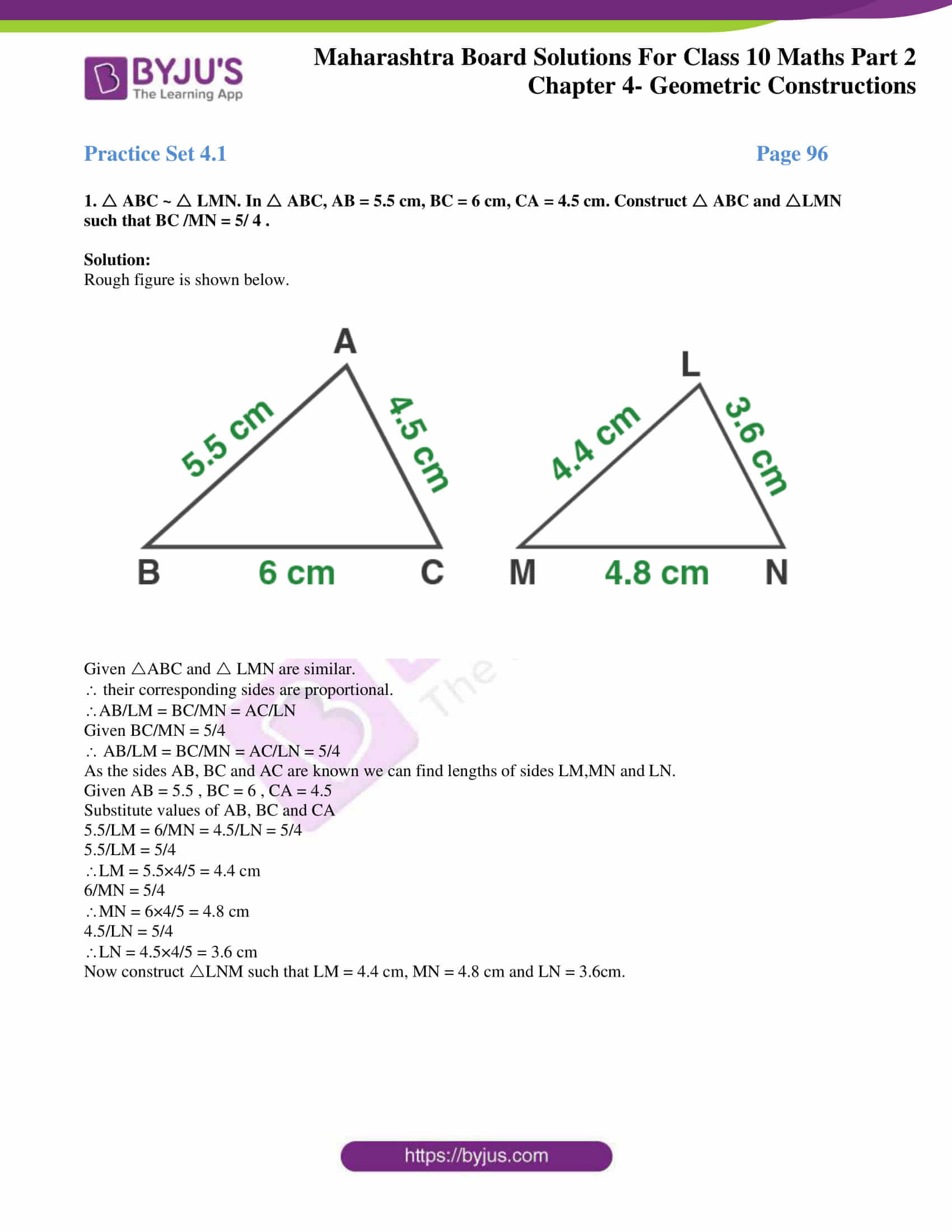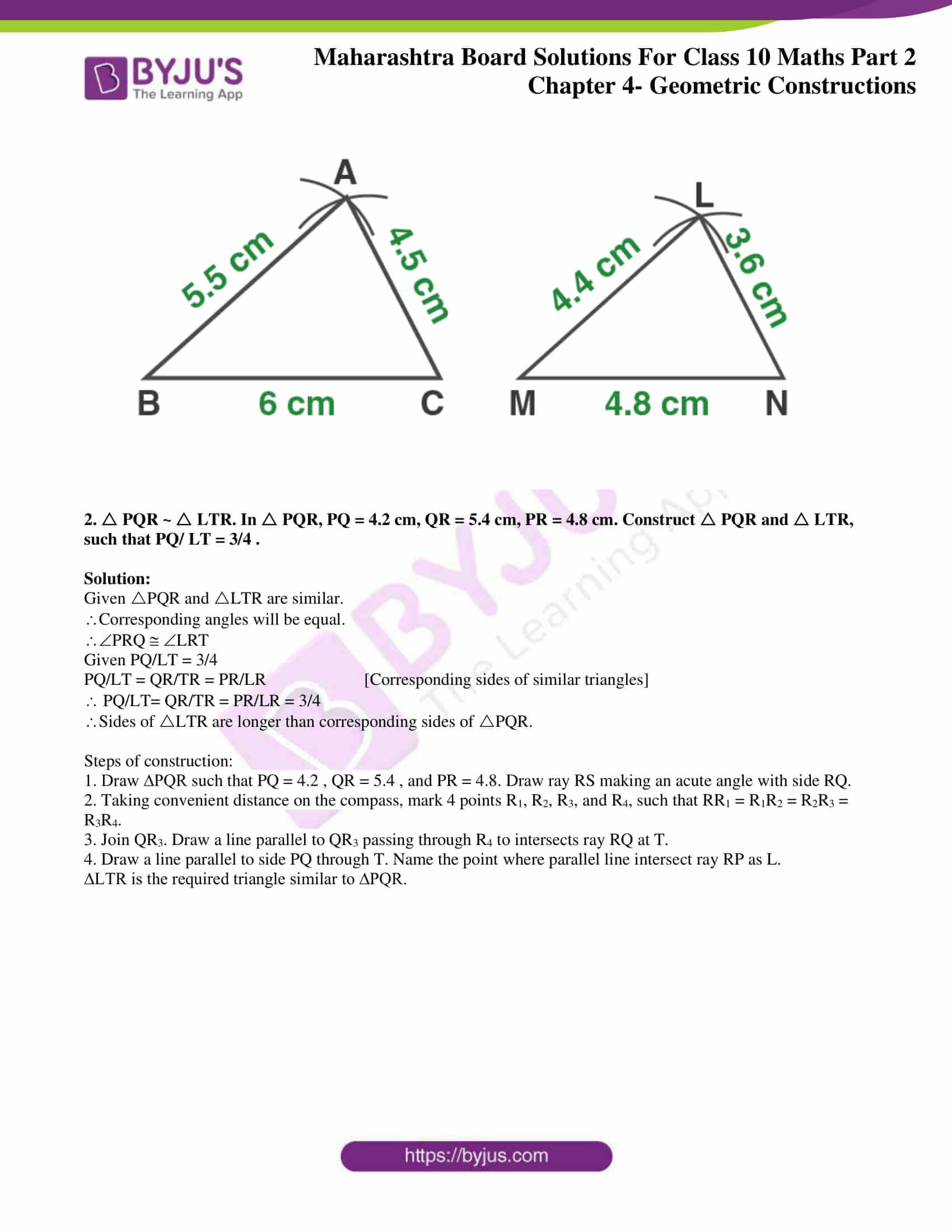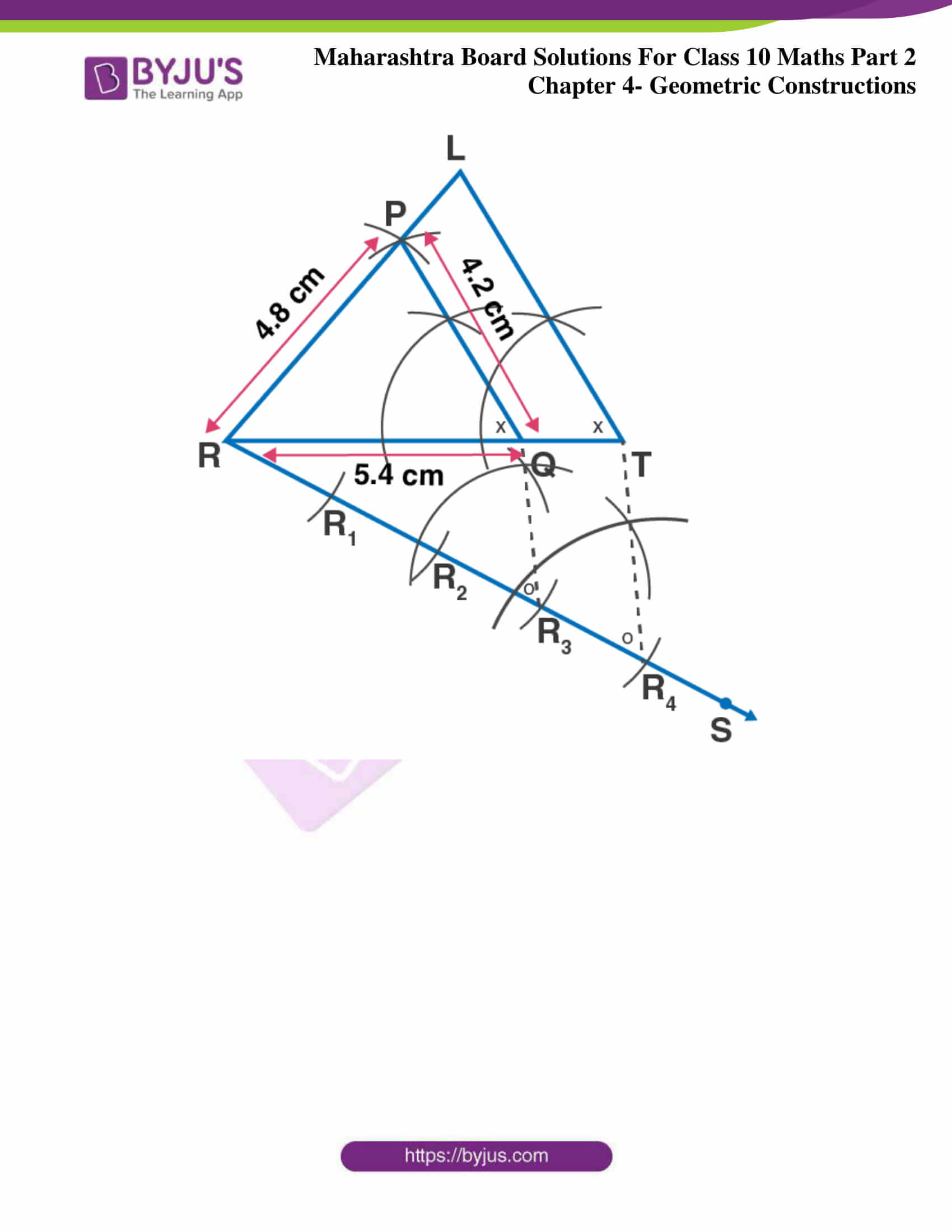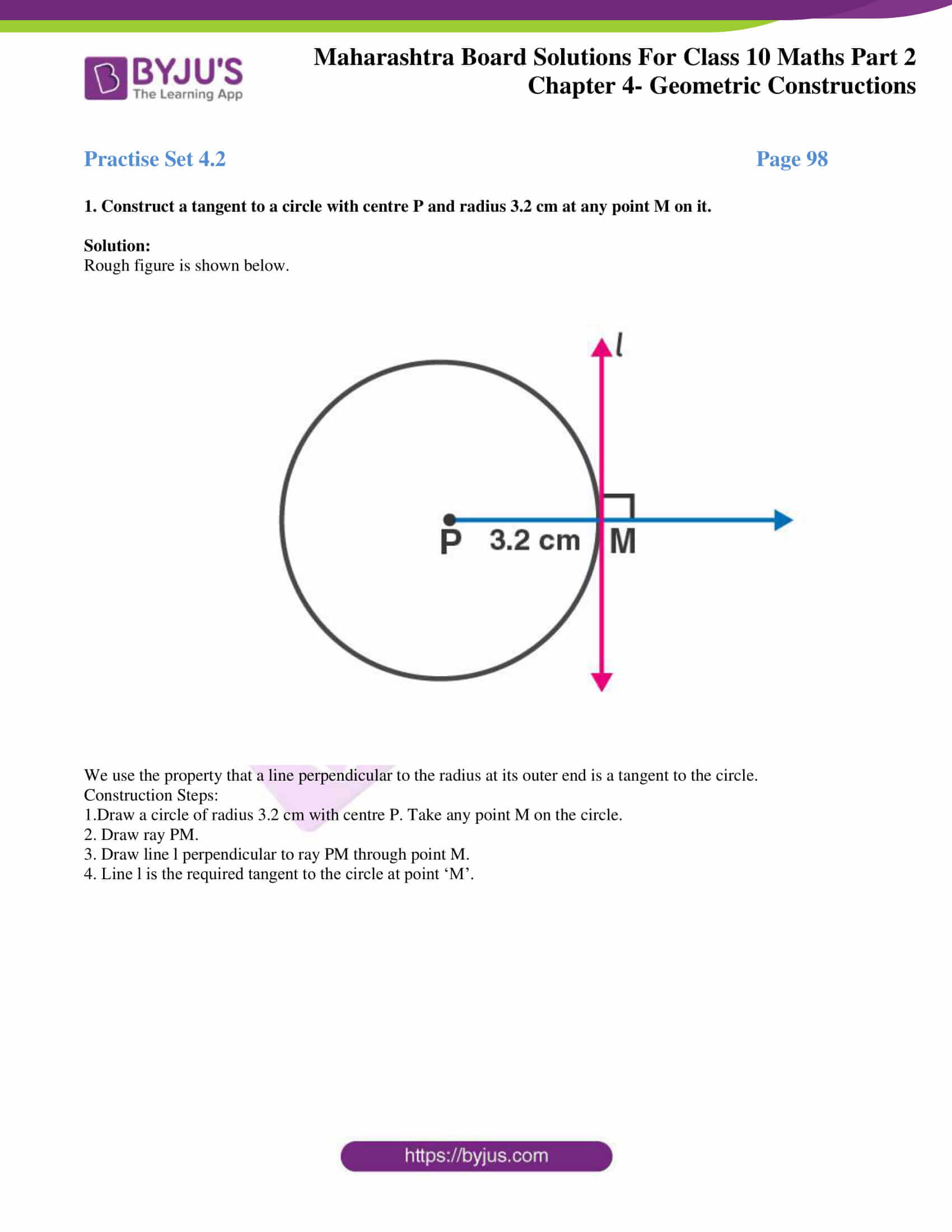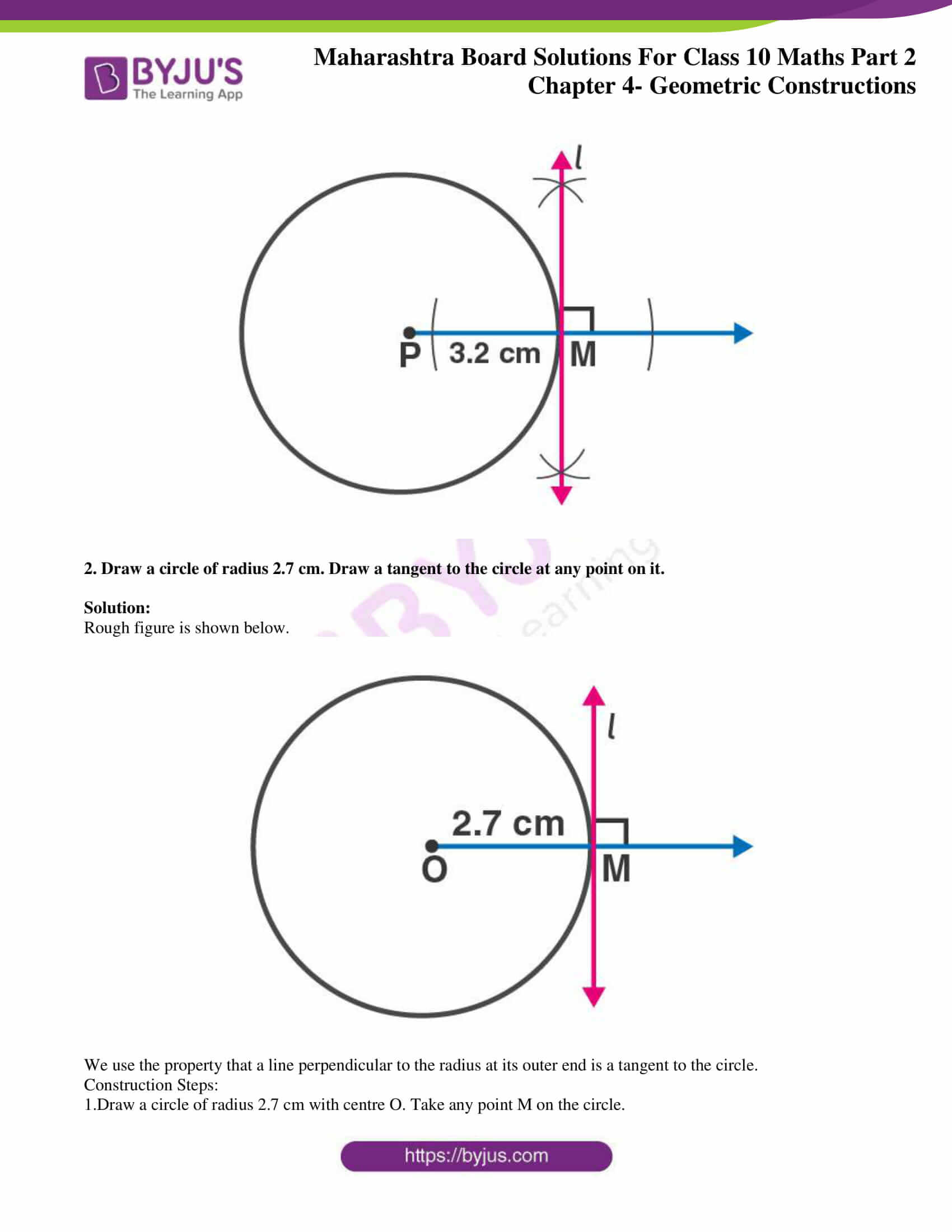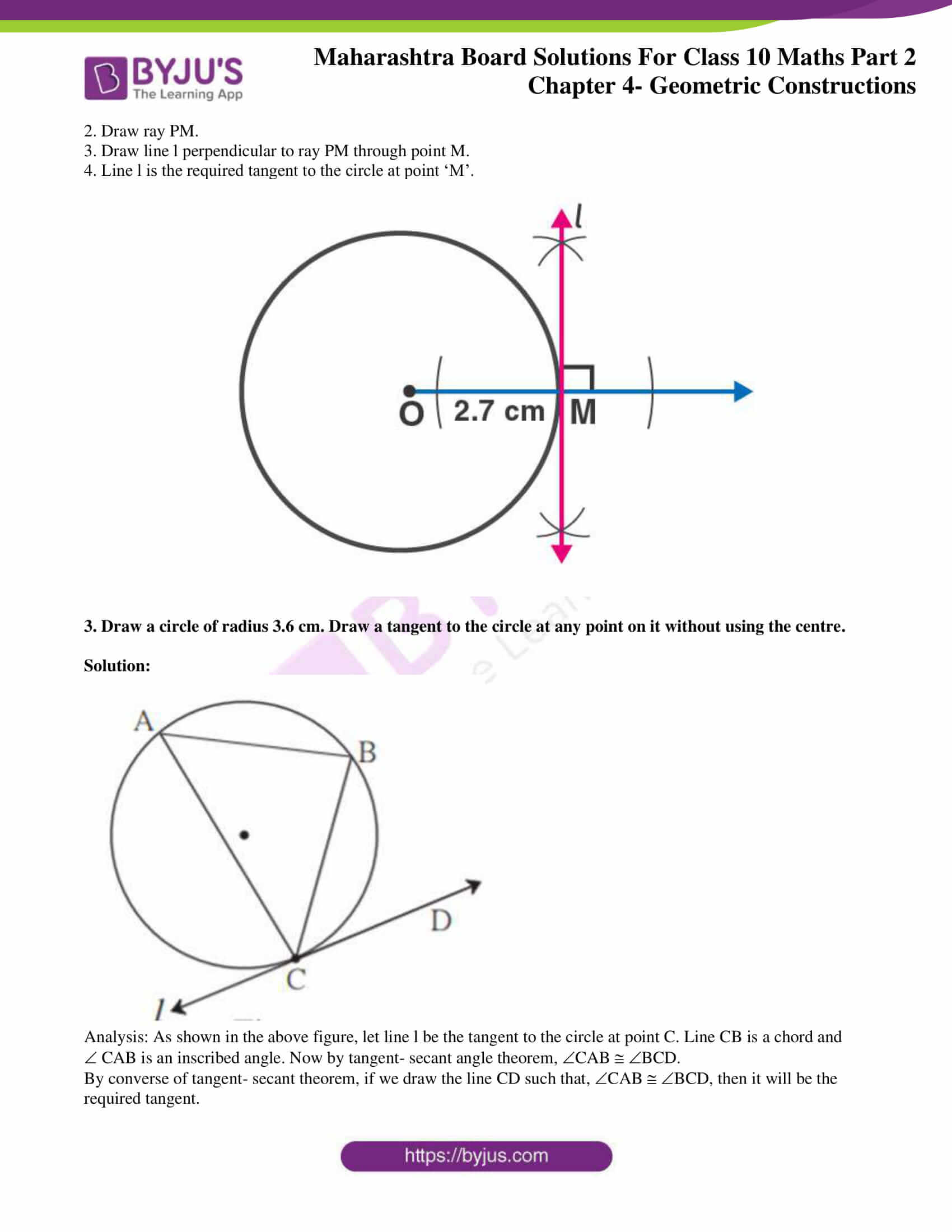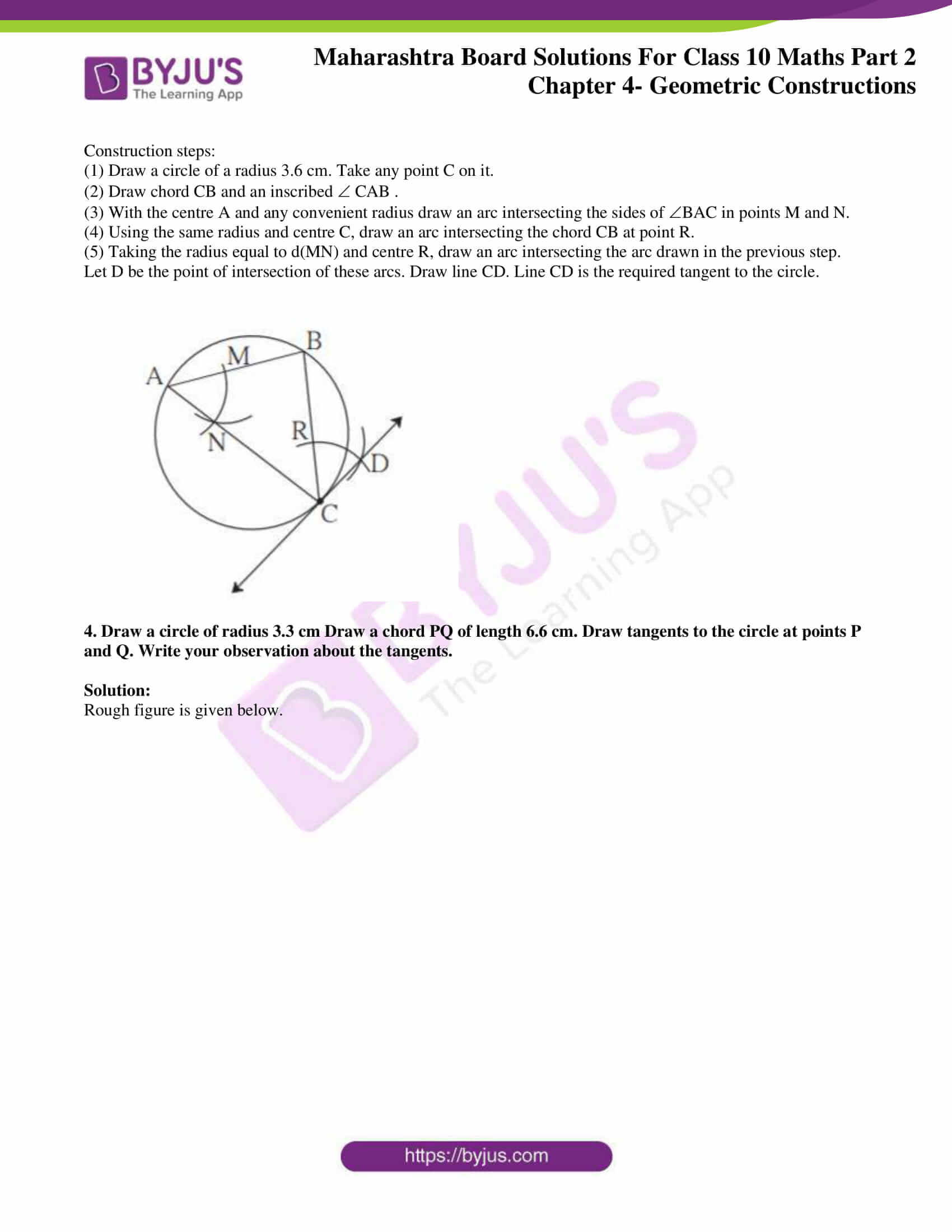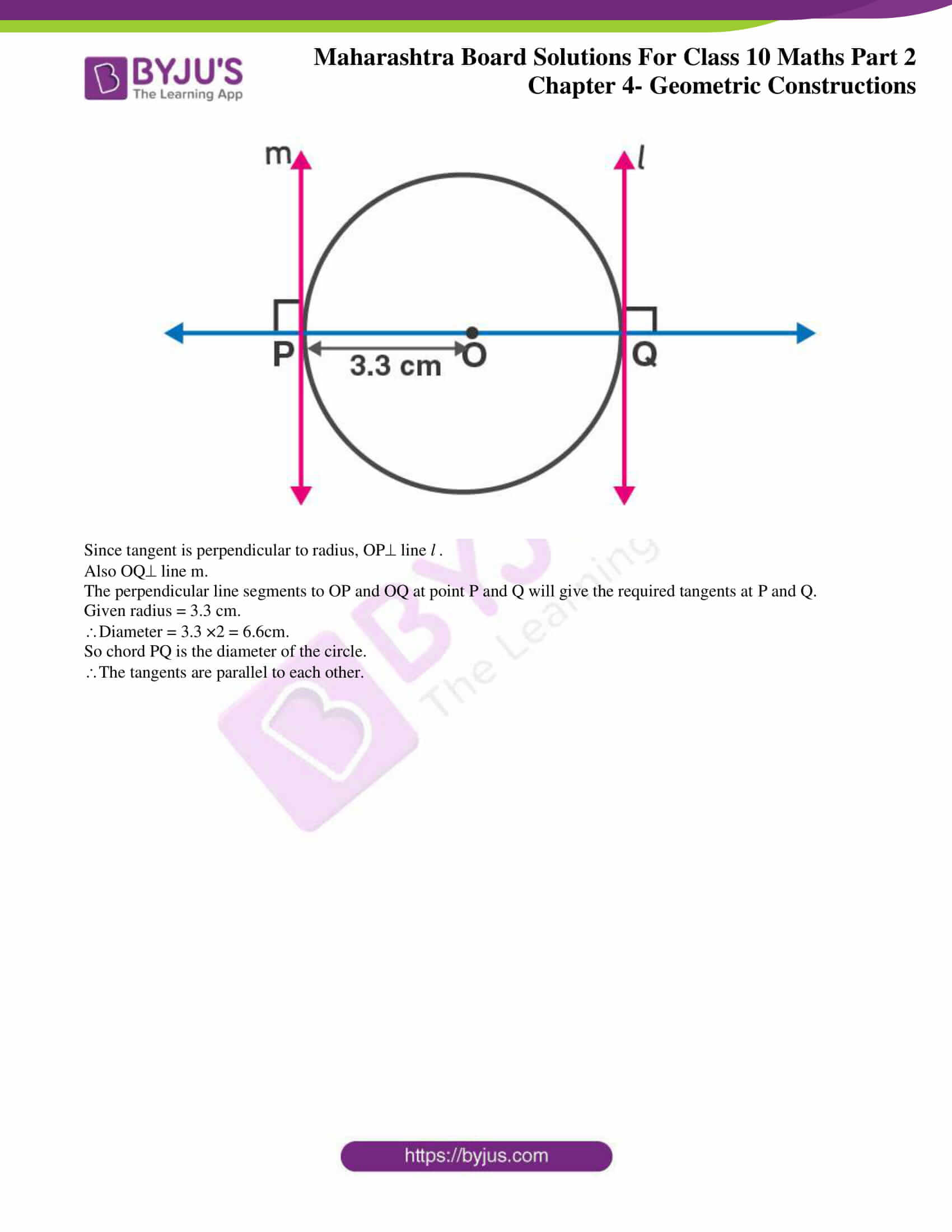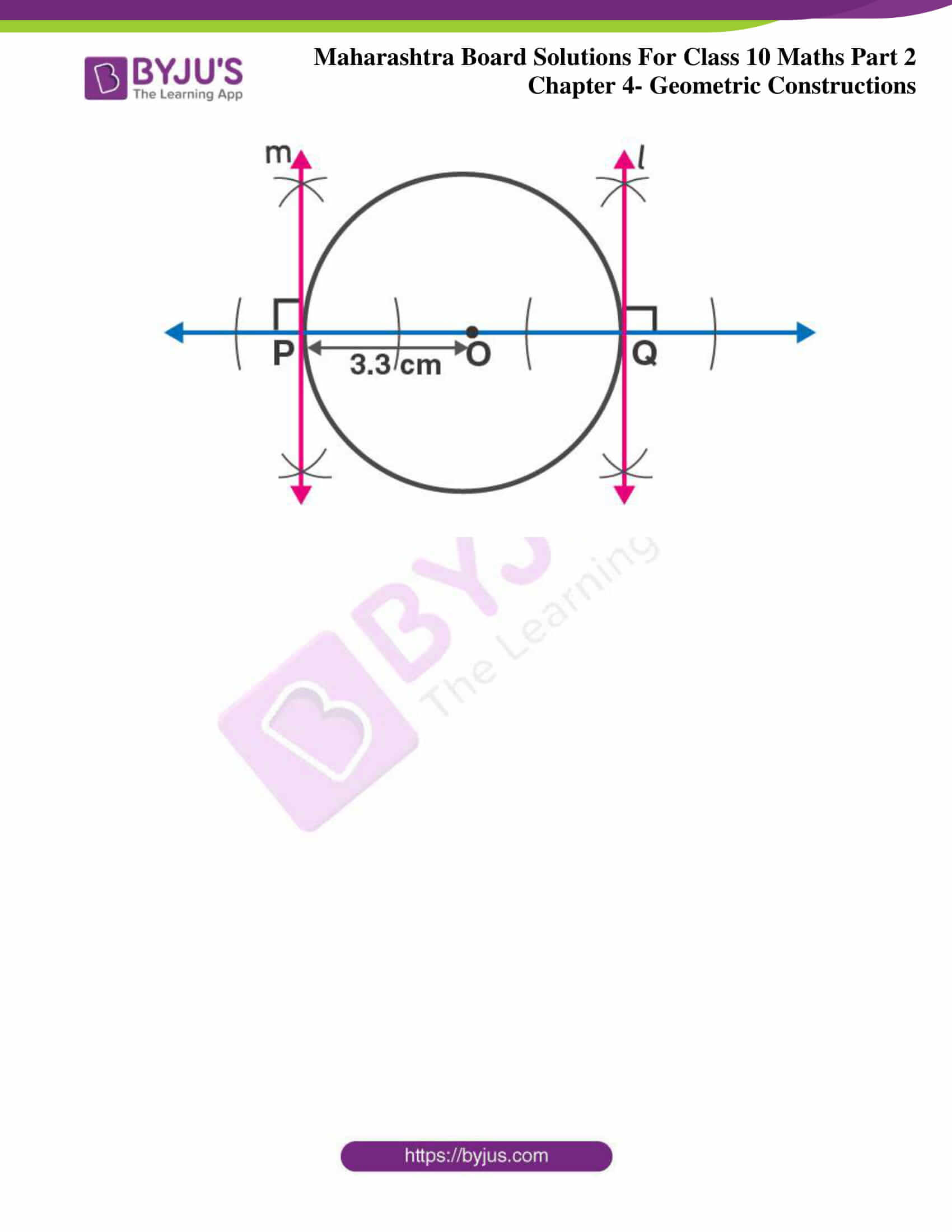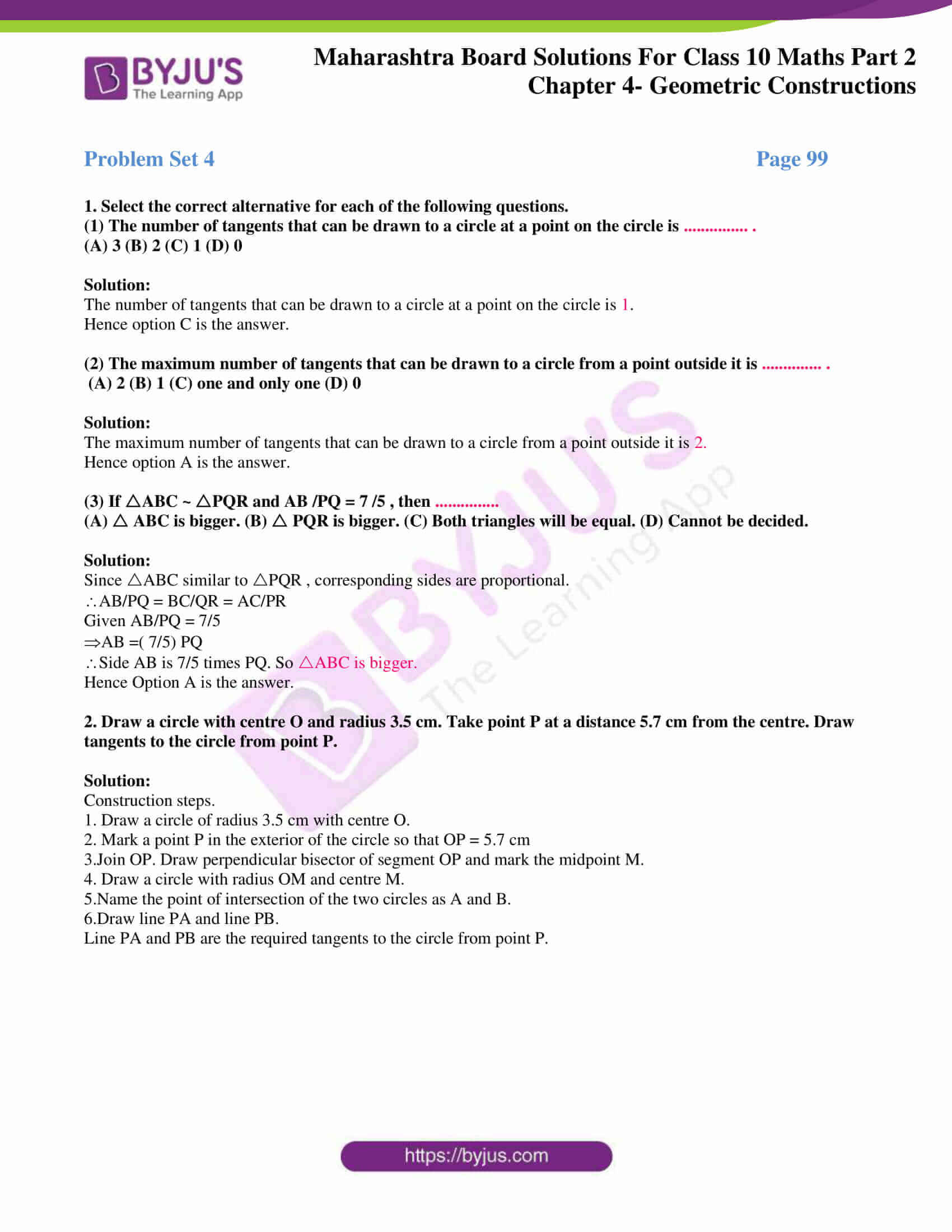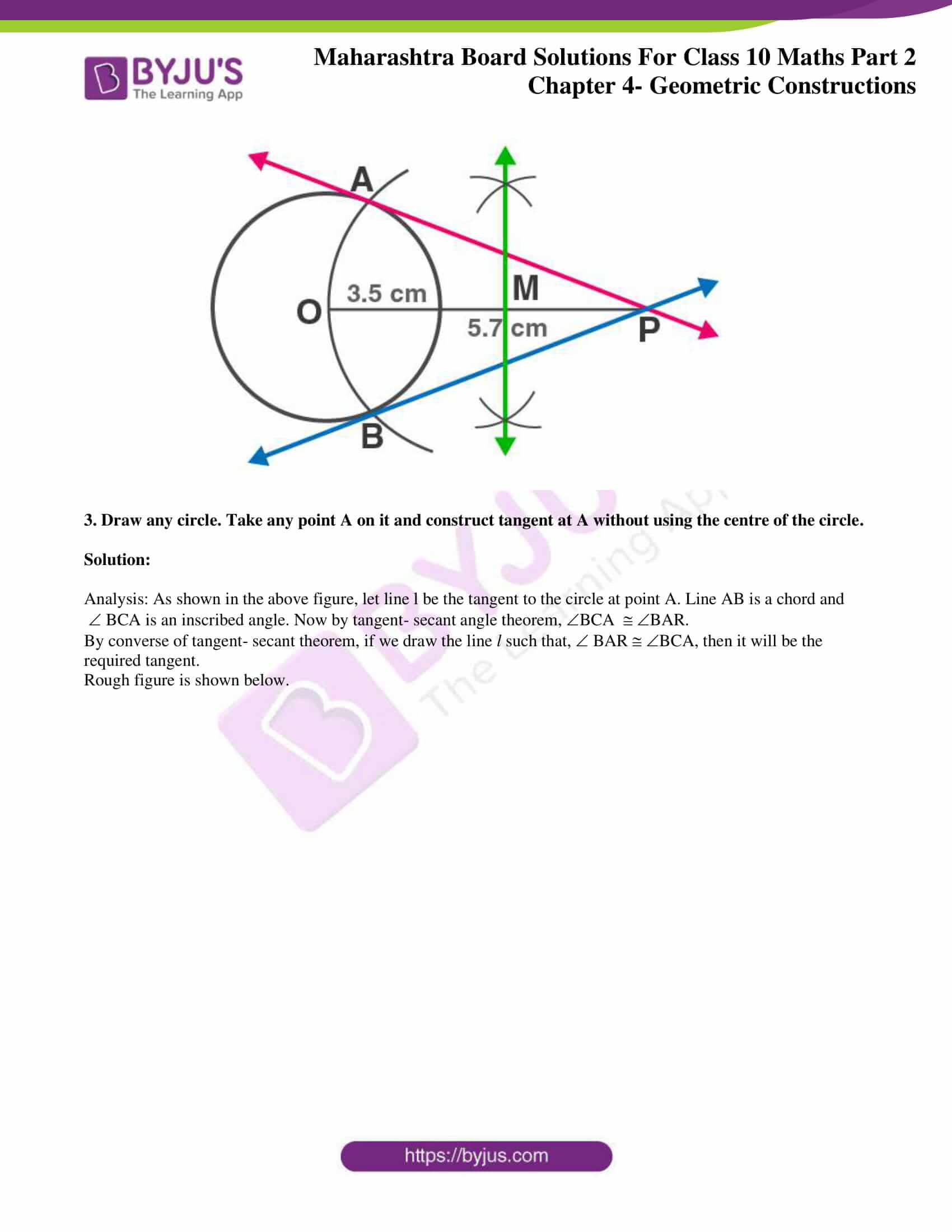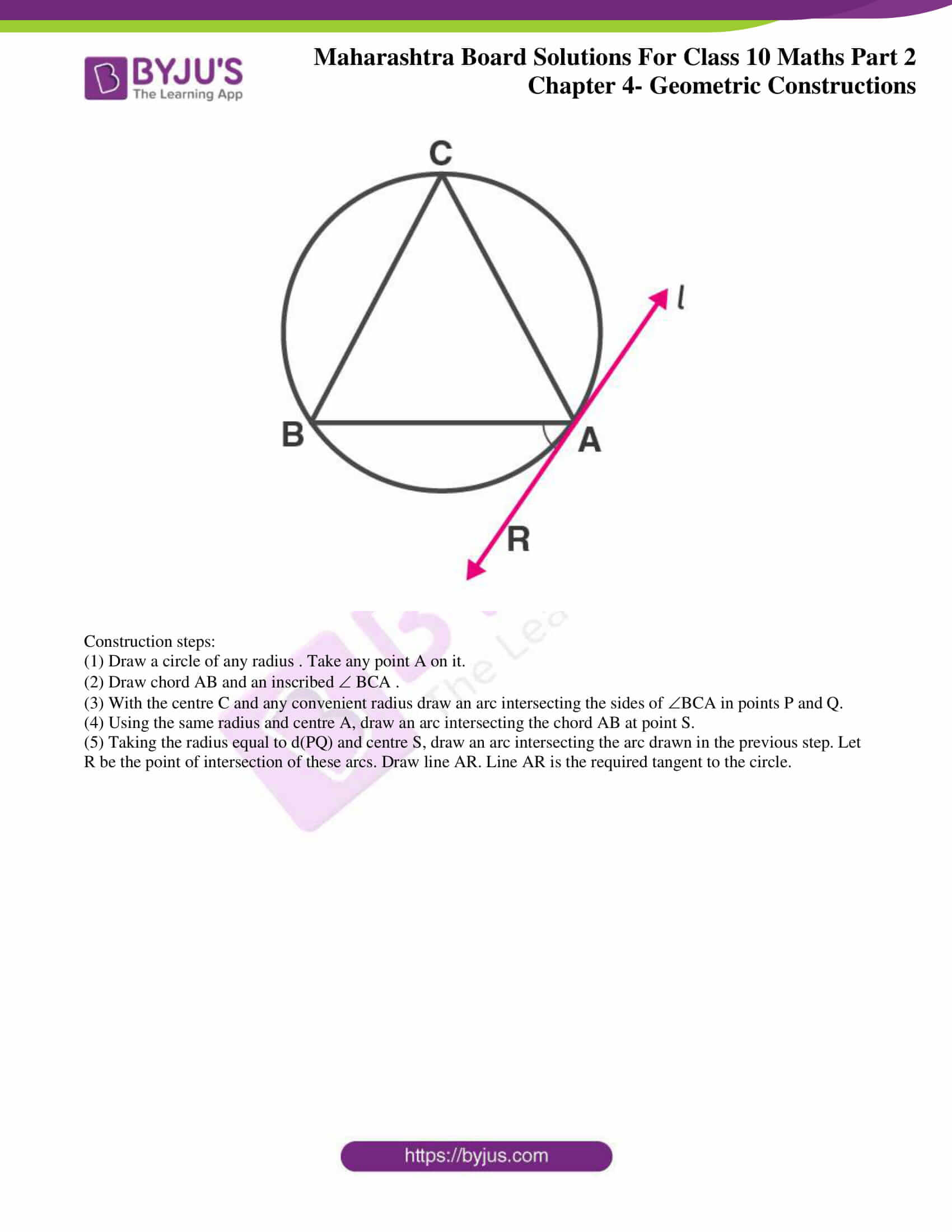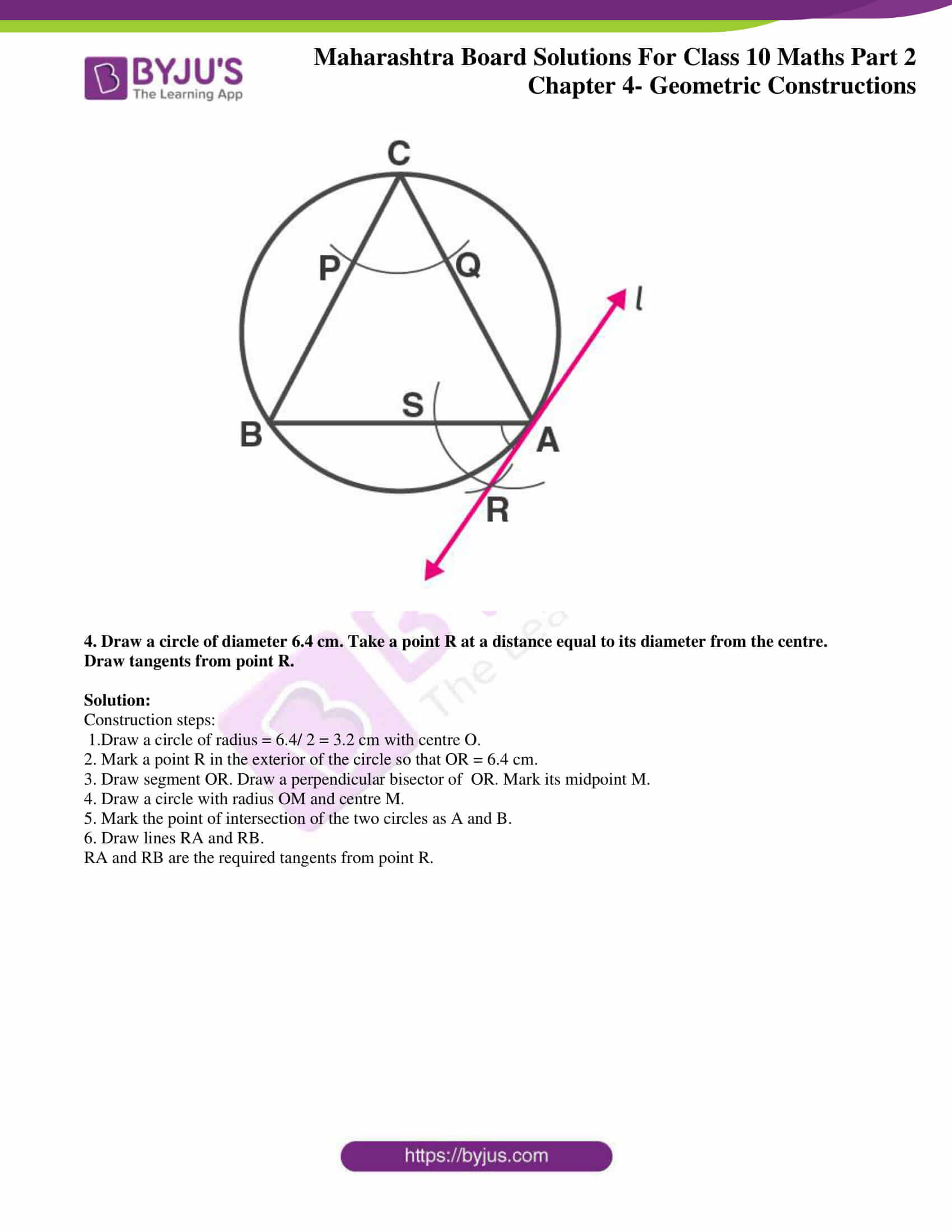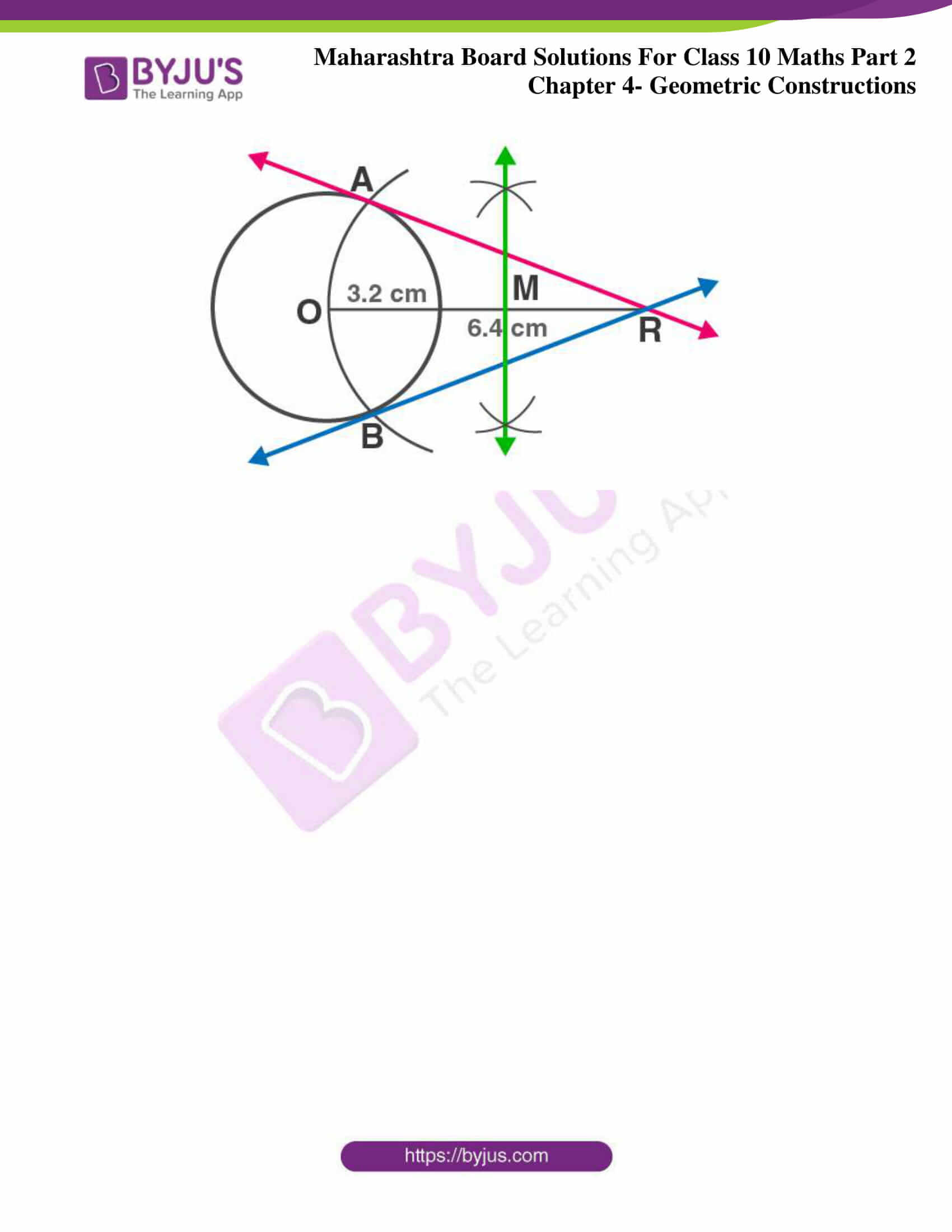## Access answers to Maths MSBSHSE Solutions For SSC Part 2 Chapter 4 – Geometric Constructions

Practice Set 4.1 Page 96

1. ABC ~ LMN. In ABC, AB = 5.5 cm, BC = 6 cm, CA = 4.5 cm. Construct ABC and LMN such that BC /MN = 5/ 4 .

Solution:

Rough figure is shown below.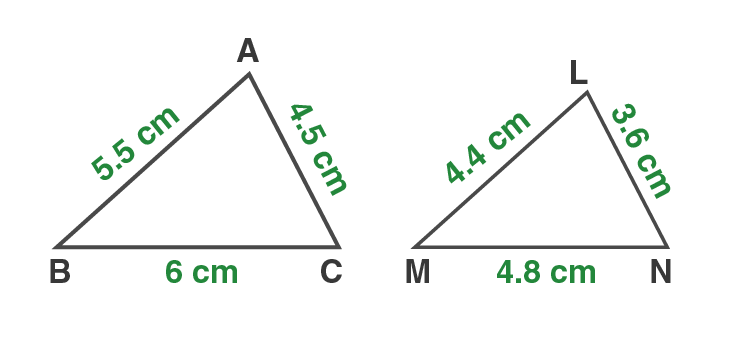Given ABC and LMN are similar.

their corresponding sides are proportional.

AB/LM = BC/MN = AC/LN

Given BC/MN = 5/4

AB/LM = BC/MN = AC/LN = 5/4

As the sides AB, BC and AC are known we can find lengths of sides LM,MN and LN.

Given AB = 5.5 , BC = 6 , CA = 4.5

Substitute values of AB, BC and CA

5.5/LM = 6/MN = 4.5/LN = 5/4

5.5/LM = 5/4

LM = 5.5×4/5 = 4.4 cm

6/MN = 5/4

MN = 6×4/5 = 4.8 cm

4.5/LN = 5/4

LN = 4.5×4/5 = 3.6 cm

Now construct LNM such that LM = 4.4 cm, MN = 4.8 cm and LN = 3.6cm.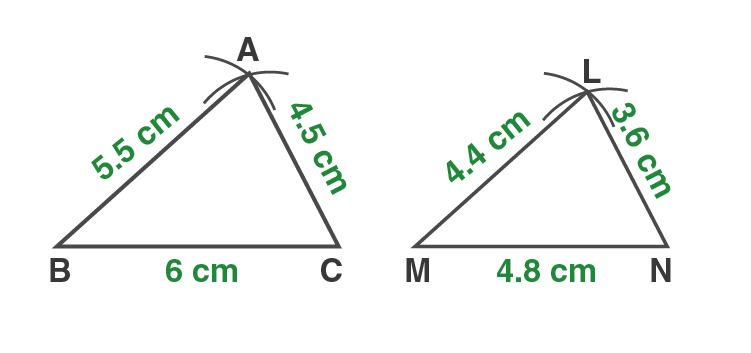2. PQR ~ LTR. In PQR, PQ = 4.2 cm, QR = 5.4 cm, PR = 4.8 cm. Construct PQR and LTR, such that PQ/ LT = 3/4 .

Solution:

Given PQR and LTR are similar.

Corresponding angles will be equal.

PRQ LRT

Given PQ/LT = 3/4

PQ/LT = QR/TR = PR/LR [Corresponding sides of similar triangles]

PQ/LT= QR/TR = PR/LR = 3/4

Sides of LTR are longer than corresponding sides of PQR.

Steps of construction:

1. Draw ∆PQR such that PQ = 4.2 , QR = 5.4 , and PR = 4.8. Draw ray RS making an acute angle with side RQ.

2. Taking convenient distance on the compass, mark 4 points R1, R2, R3, and R4, such that RR1 = R1R2 = R2R3 = R3R4.

3. Join QR3. Draw a line parallel to QR3 passing through R4 to intersects ray RQ at T.

4. Draw a line parallel to side PQ through T. Name the point where parallel line intersect ray RP as L.

∆LTR is the required triangle similar to ∆PQR.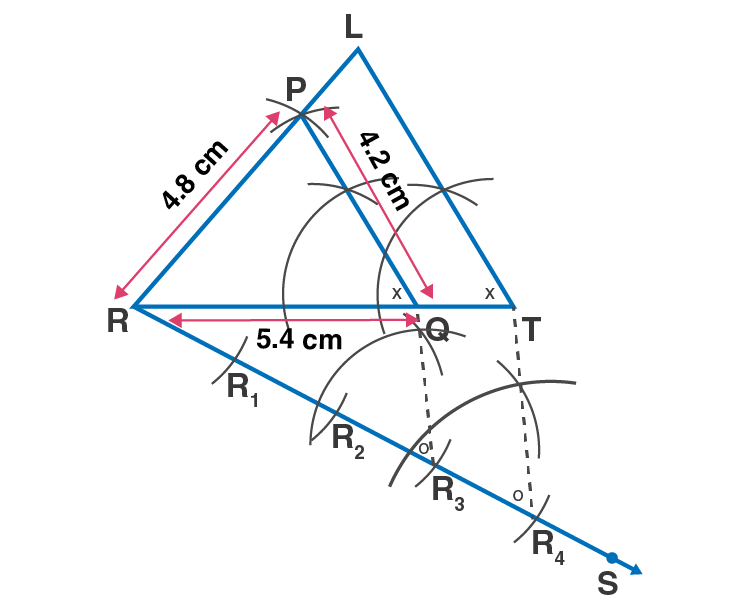Practise Set 4.2 Page 98

1. Construct a tangent to a circle with centre P and radius 3.2 cm at any point M on it.

Solution:

Rough figure is shown below.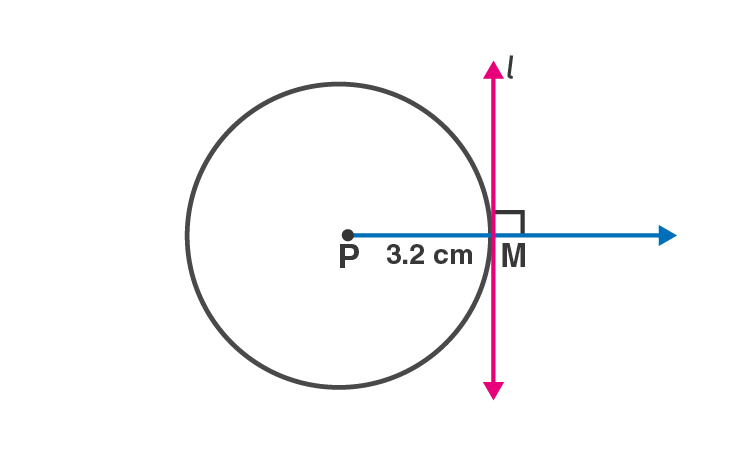We use the property that a line perpendicular to the radius at its outer end is a tangent to the circle.

Construction Steps:

1.Draw a circle of radius 3.2 cm with centre P. Take any point M on the circle.

2. Draw ray PM.

3. Draw line l perpendicular to ray PM through point M.

4. Line l is the required tangent to the circle at point ‘M’.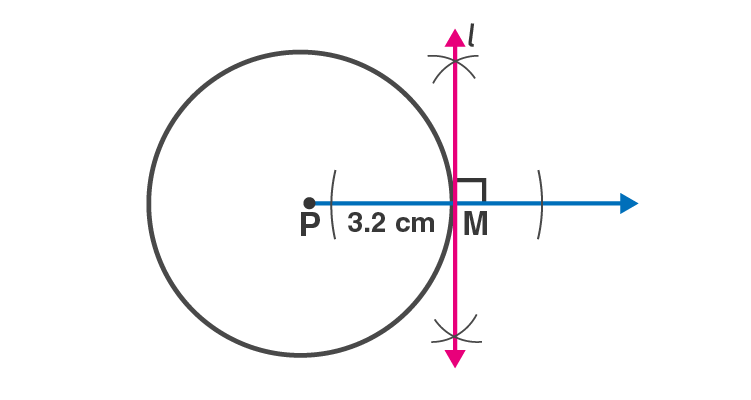2. Draw a circle of radius 2.7 cm. Draw a tangent to the circle at any point on it.

Solution:

Rough figure is shown below.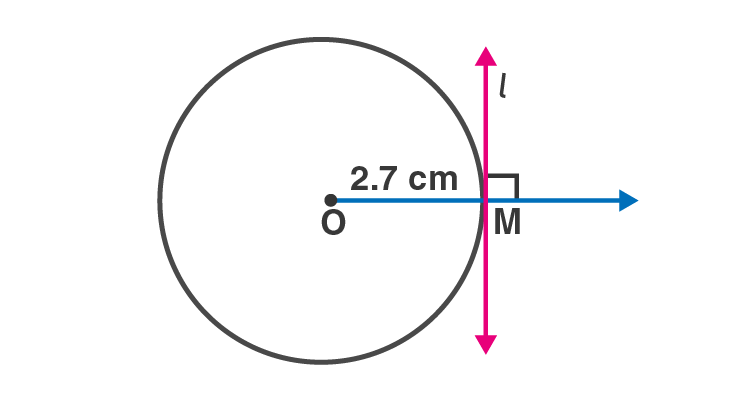We use the property that a line perpendicular to the radius at its outer end is a tangent to the circle.

Construction Steps:

1.Draw a circle of radius 2.7 cm with centre O. Take any point M on the circle.

2. Draw ray PM.

3. Draw line l perpendicular to ray PM through point M.

4. Line l is the required tangent to the circle at point ‘M’.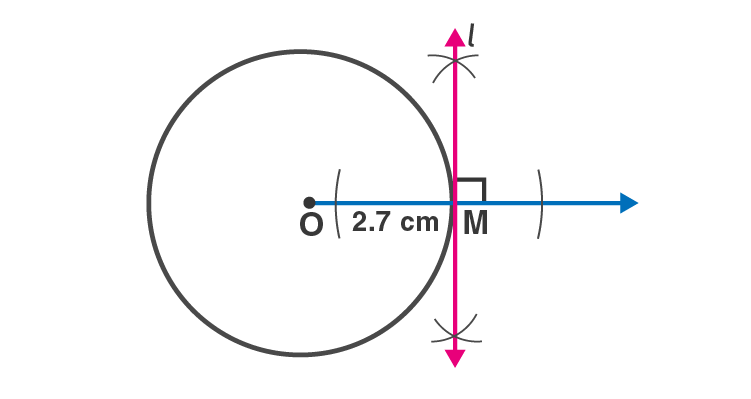3. Draw a circle of radius 3.6 cm. Draw a tangent to the circle at any point on it without using the centre.

Solution: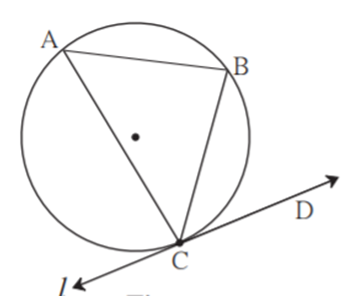Analysis: As shown in the above figure, let line l be the tangent to the circle at point C. Line CB is a chord and

CAB is an inscribed angle. Now by tangent- secant angle theorem, CAB BCD.

By converse of tangent- secant theorem, if we draw the line CD such that, CAB BCD, then it will be the required tangent.

Construction steps:

(1) Draw a circle of a radius 3.6 cm. Take any point C on it.

(2) Draw chord CB and an inscribed CAB .

(3) With the centre A and any convenient radius draw an arc intersecting the sides of BAC in points M and N. (4) Using the same radius and centre C, draw an arc intersecting the chord CB at point R.

(5) Taking the radius equal to d(MN) and centre R, draw an arc intersecting the arc drawn in the previous step. Let D be the point of intersection of these arcs. Draw line CD. Line CD is the required tangent to the circle.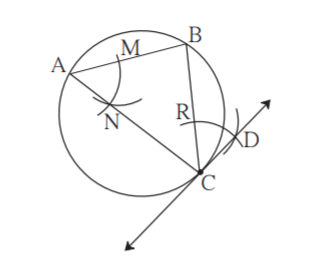4. Draw a circle of radius 3.3 cm Draw a chord PQ of length 6.6 cm. Draw tangents to the circle at points P and Q. Write your observation about the tangents.

Solution:

Rough figure is given below.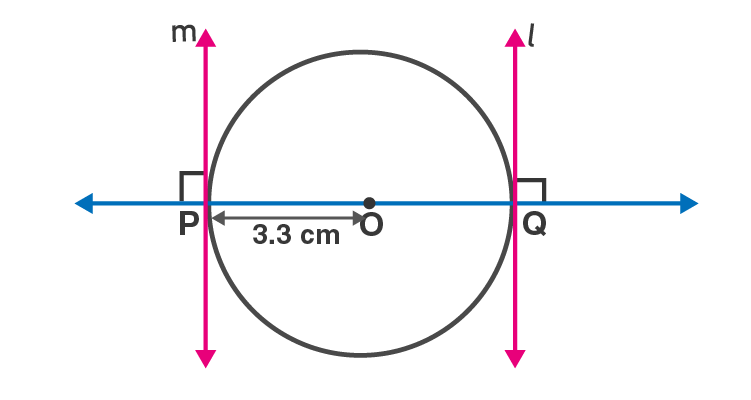Since tangent is perpendicular to radius, OP line l .

Also OQ line m.

The perpendicular line segments to OP and OQ at point P and Q will give the required tangents at P and Q.

Diameter = 3.3 ×2 = 6.6cm.

So chord PQ is the diameter of the circle.

The tangents are parallel to each other.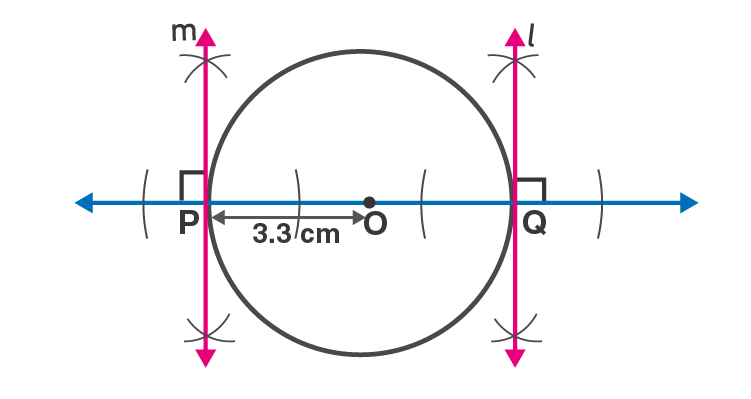Problem Set 4 Page 99

1. Select the correct alternative for each of the following questions.

(1) The number of tangents that can be drawn to a circle at a point on the circle is …………… .

(A) 3 (B) 2 (C) 1 (D) 0

Solution:

The number of tangents that can be drawn to a circle at a point on the circle is 1.

Hence option C is the answer.

(2) The maximum number of tangents that can be drawn to a circle from a point outside it is ………….. .

(A) 2 (B) 1 (C) one and only one (D) 0

Solution:

The maximum number of tangents that can be drawn to a circle from a point outside it is 2.

Hence option A is the answer.

(3) If ABC ~ PQR and AB /PQ = 7 /5 , then ……………

(A) ABC is bigger. (B) PQR is bigger. (C) Both triangles will be equal. (D) Cannot be decided.

Solution:

Since ABC similar to PQR , corresponding sides are proportional.

AB/PQ = BC/QR = AC/PR

Given AB/PQ = 7/5

AB =( 7/5) PQ

Side AB is 7/5 times PQ. So ABC is bigger.

Hence Option A is the answer.

2. Draw a circle with centre O and radius 3.5 cm. Take point P at a distance 5.7 cm from the centre. Draw tangents to the circle from point P.

Solution:

Construction steps.

1. Draw a circle of radius 3.5 cm with centre O.

2. Mark a point P in the exterior of the circle so that OP = 5.7 cm

3.Join OP. Draw perpendicular bisector of segment OP and mark the midpoint M.

4. Draw a circle with radius OM and centre M.

5.Name the point of intersection of the two circles as A and B.

6.Draw line PA and line PB.

Line PA and PB are the required tangents to the circle from point P.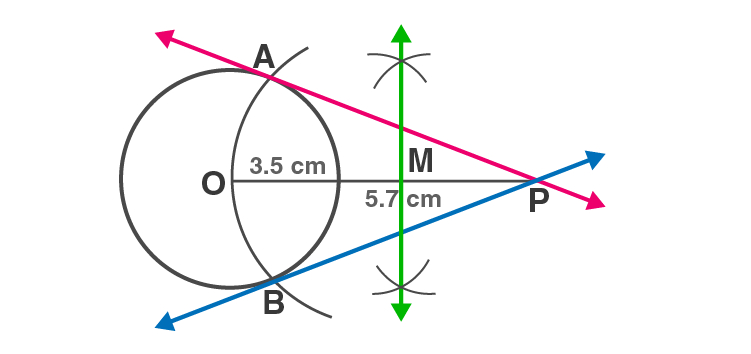3. Draw any circle. Take any point A on it and construct tangent at A without using the centre of the circle.

Solution:

Analysis: As shown in the above figure, let line l be the tangent to the circle at point A. Line AB is a chord and

BCA is an inscribed angle. Now by tangent- secant angle theorem, BCA BAR.

By converse of tangent- secant theorem, if we draw the line l such that, BAR BCA, then it will be the required tangent.

Rough figure is shown below.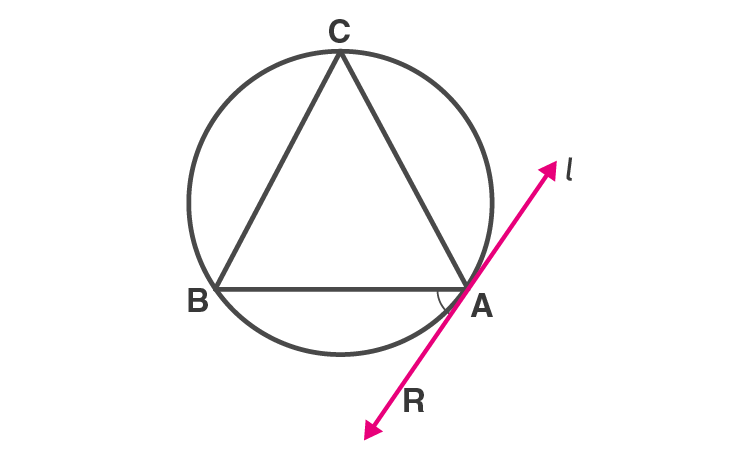Construction steps:

(1) Draw a circle of any radius . Take any point A on it.

(2) Draw chord AB and an inscribed BCA .

(3) With the centre C and any convenient radius draw an arc intersecting the sides of BCA in points P and Q.

(4) Using the same radius and centre A, draw an arc intersecting the chord AB at point S.

(5) Taking the radius equal to d(PQ) and centre S, draw an arc intersecting the arc drawn in the previous step. Let R be the point of intersection of these arcs. Draw line AR. Line AR is the required tangent to the circle.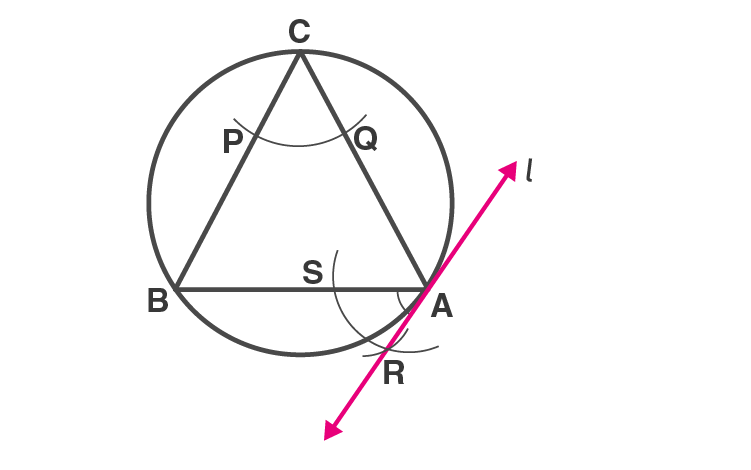4. Draw a circle of diameter 6.4 cm. Take a point R at a distance equal to its diameter from the centre. Draw tangents from point R.

Solution:

Construction steps:

1.Draw a circle of radius = 6.4/ 2 = 3.2 cm with centre O.

2. Mark a point R in the exterior of the circle so that OR = 6.4 cm.

3. Draw segment OR. Draw a perpendicular bisector of OR. Mark its midpoint M.

4. Draw a circle with radius OM and centre M.

5. Mark the point of intersection of the two circles as A and B.

6. Draw lines RA and RB.

RA and RB are the required tangents from point R.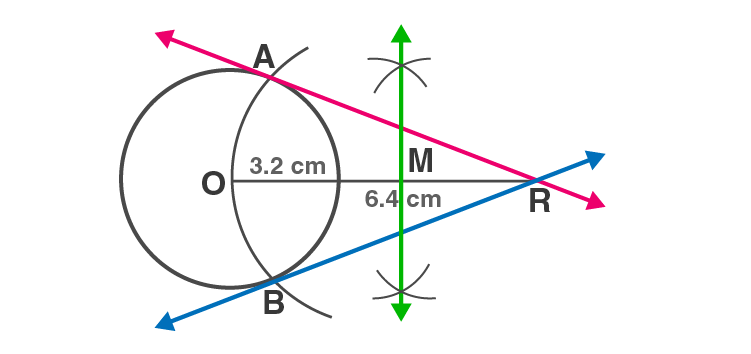Geometric Constructions includes drawing of similar triangles and tangents to a circle. Construction of tangents to a given circle from a point outside the circle is also explained in this chapter. These solutions help students to improve their confidence to crack the problems for examinations.

## Frequently Asked Questions on Maharashtra State Board Solutions for Class 10 Maths Part 2 Chapter 4 Geometric Constructions

### What are the concepts explained in Maharashtra State Board Class 10 Maths Part 2 Chapter 4 Solutions?

These solutions help students to familiarize themselves with geometric constructions of triangles, similar to a given circle. This chapter also deals with construction of tangents to a circle using the centre of the circle and without using the centre of the circle.

### Are these Maharashtra State Board Class 10 Maths Part 2 Chapter 4 Solutions helpful?

Yes, these solutions are very helpful. Students are encouraged to practise these questions at first. Then they can refer back to the solutions to analyse their performance. This will also help them to rectify the mistakes, so that they can avoid making any during the board exams. These solutions also lay the foundation for board exam questions.

### How can we access these solutions?

It is possible to download the solutions. We have provided the solutions as a scrollable PDF, and we have also mentioned the clickable link for the students to access. Meanwhile, we have also made these questions and the solutions available online on our webpage.